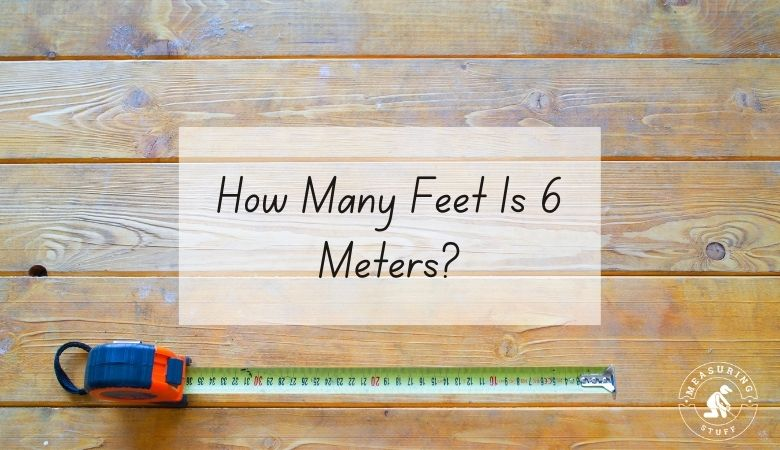# How Many Feet Is 6 Meters?

Figuring out how many feet are in 6 meters might seem like a challenge at first, but a simple math equation can help you figure it out easily.

Six meters is equal to about 19.658 feet.

We know this because there are about 3.281 feet to every 1 meter.

Using this information, you can find out how many feet are in a given number of meters by multiplying the number of meters by the conversion factor of 3.281.

6 meters x 3.281 (conversion factor) = 19.658.

For another example, if you want to know how many feet are in 10 meters, you would multiply 10 times 3.281 to get 32.81, which is approximately how many feet there are in 10 meters.

This works for smaller numbers, too. If you want to know how many feet are in 2 meters, multiply 2 by 3.281 to get about 6.56.

You can also reverse this equation in order to find out how many meters you can make out of a given number of feet.

To do this, you’ll need to divide the number of feet by 3.281.

For example, if you have 12 feet and want to know how many meters that equals, you can divide 12 by 3.281 to get about 3.66, which is how many meters you can make from 12 feet.

If you don’t feel like doing the math, use our online conversion calculator to convert different units of measurements and quickly give you the answer.

## Units of measurement conversion calculator

Multiplying or dividing by such an odd number can sometimes be a bit tricky, especially if you’re trying to do it as you work.

For this reason, you might find it simpler to use a conversion chart to help you calculate how many feet are in a given number of meters or how many meters you can make from a given number of feet.

## Feet into meters conversion table

When you’re converting feet into meters or vice versa, it may also help you to remember that a meter is almost the same length as a yard.

A yard is exactly 3 feet or 36 inches long. A single meter is just a tiny bit shorter than this length.

Feet are a measurement of length in the imperial system, which is widely used in the United States.

Meters are part of the metric system, which is a base-ten system that’s used around the world.

## Related articles:

Check out a complete list of related articles in this category here.